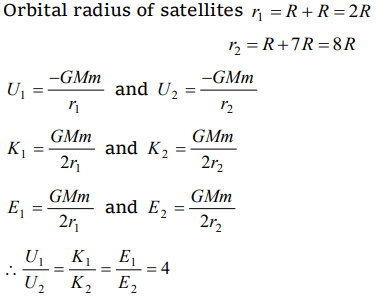## Gravitation Questions and Answers Part-20

1. The orbital velocity of an artificial satellite in a circular orbit just above the earth's surface is v. For a satellite orbiting at an altitude of half of the earth's radius, the orbital velocity is
a) $\frac{3}{2}v$
b) $\sqrt{\frac{3}{2}}v$
c) $\sqrt{\frac{2}{3}}v$
d) $\frac{2}{3}v$

Explanation:2. In a satellite if the time of revolution is T, then K.E. is proportional to
a) $\frac{1}{T}$
b) $\frac{1}{T^{2}}$
c) $\frac{1}{T^{3}}$
d) $\frac{1}{T^{2/3}}$

Explanation: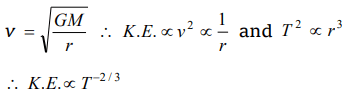3. If the height of a satellite from the earth is negligible in comparison to the radius of the earth R, the orbital velocity of the satellite is
a) gR
b) gR/2
c) $\sqrt{g/R}$
d) $\sqrt{gR}$

Explanation: $\sqrt{gR}$

4. Choose the correct statement from the following : The radius of the orbit of a geostationary satellite depends upon
a) Mass of the satellite, its time period and the gravitational constant
b) Mass of the satellite, mass of the earth and the gravitational constant
c) Mass of the earth, mass of the satellite, time period of the satellite and the gravitational constant
d) Mass of the earth, time period of the satellite and the gravitational constant

Explanation: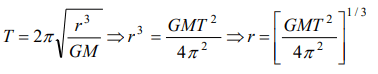5. Out of the following, the only incorrect statement about satellites is
a) A satellite cannot move in a stable orbit in a plane passing through the earth's centre
b) Geostationary satellites are launched in the equatorial plane
c) We can use just one geostationary satellite for global communication around the globe
d) The speed of a satellite increases with an increase in the radius of its orbit

Explanation: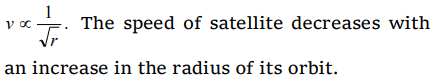6. A satellite is moving around the earth with speed v in a circular orbit of radius r. If the orbit radius is decreased by 1%, its speed will
a) Increase by 1%
b) Increase by 0.5%
c) Decrease by 1%
d) Decrease by 0.5%

Explanation: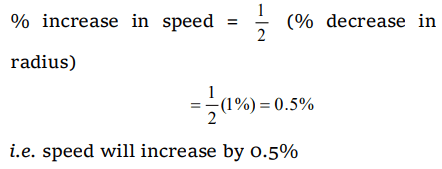7. Orbital velocity of an artificial satellite does not depend upon
a) Mass of the earth
b) Mass of the satellite
d) Acceleration due to gravity

Explanation: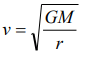8. The time period of a geostationary satellite is
a) 24 hours
b) 12 hours
c) 365 days
d) One month

Explanation: The time period of a geostationary satellite is 24 hours

9. Orbital velocity of earth's satellite near the surface is 7 km/s. When the radius of the orbit is 4 times than that of earth's radius, then orbital velocity in that orbit is
a) 3.5 km/s
b) 7 km/s
c) 72 km/s
d) 14 km/s